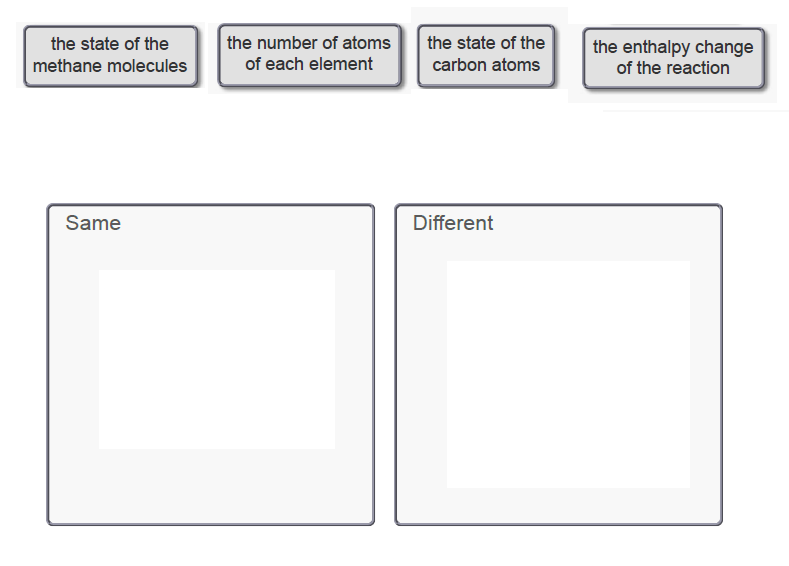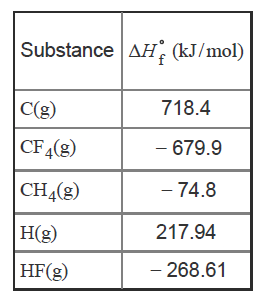# Problem: Compare the reaction for the "expansion" of methane with the reverse of the reaction that represents the standard enthalpy of formation. Which properties are the same for both reactions and which are different?Drag each item to the appropriate bin.Use the data below to answer the questions.Keep in mind that the enthalpy of formation of an element in its standard state is zero.Imagine a hypothetical process in which the methane molecule, CH4, is "expanded," by simultaneously extending all four C−H bonds to infinity. We then have the processCH4(g) → C(g) + 4 H(g)

###### FREE Expert Solution
83% (123 ratings)View Complete Written Solution
###### Problem Details

Compare the reaction for the "expansion" of methane with the reverse of the reaction that represents the standard enthalpy of formation. Which properties are the same for both reactions and which are different?

Drag each item to the appropriate bin.Use the data below to answer the questions.Keep in mind that the enthalpy of formation of an element in its standard state is zero.

Imagine a hypothetical process in which the methane molecule, CH4, is "expanded," by simultaneously extending all four C−H bonds to infinity. We then have the process

CH4(g) → C(g) + 4 H(g)